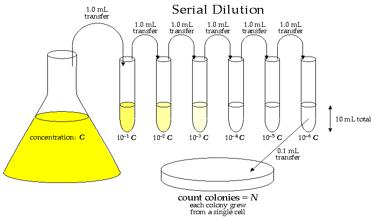Chemistry

# What is Diluting Solutions?

Often, in analytical work, it is necessary to know how to prepare a more dilute solution from a more concentrated one. This process is called Dilution. To dilute a solution, water must be added. When extra water (the solvent) is added, the number of moles of solute does not change, but the volume of the solution does change, hence the concentration changes.

For example, solution A has been diluted twice. Which of these quantities remain constant:

• volume,
• concentration,
• number of moles?A very useful equation is: C original x V original = C diluted x V diluted

Where:

• C original and V original are the concentration and volume of original solution,
• C diluted and V diluted are the concentration and volume of diluted solution

Often written as CI x V1 = C2 x V2. When using this equation, the units of concentration and volume must be the same on both sides.

Example

If you have a solution containing 10 g.L-1 of hydrochloric acid, how would you prepare 100 cm3 of a 0.1 M solution? (R.A.M. H = 1.0, Cl = 35.5)

Part 1: calculate the molarity of the original solution:

R.M.M. of HCI = 36.5 g mol-1 so concentration of original solution = 0.274 M

Part 2: the dilution

C original x V original = C dilute x V dilute

0.274 x V original = 0.1 x 100

V original = 0.1 x 100 /0.274 = 36.5 cm3

So measure carefully 36.5 cm3 of the original solution into a 100 cm3 volumetric flask and make it up to 100 cm3 with distilled water.